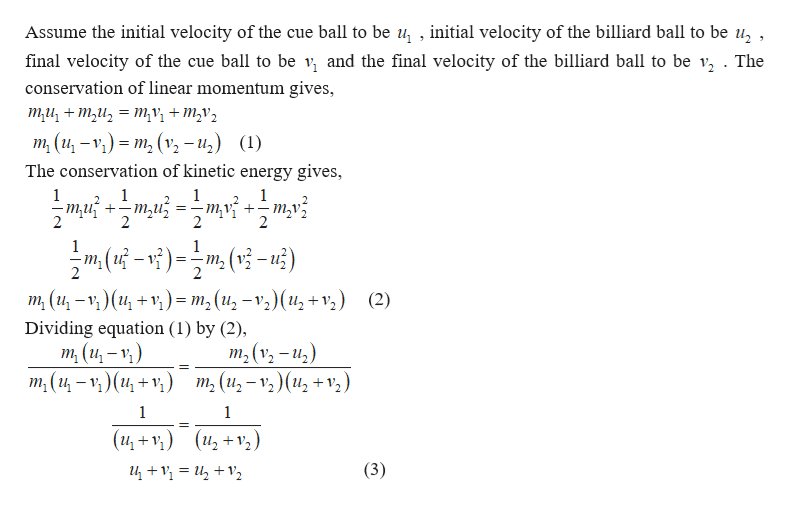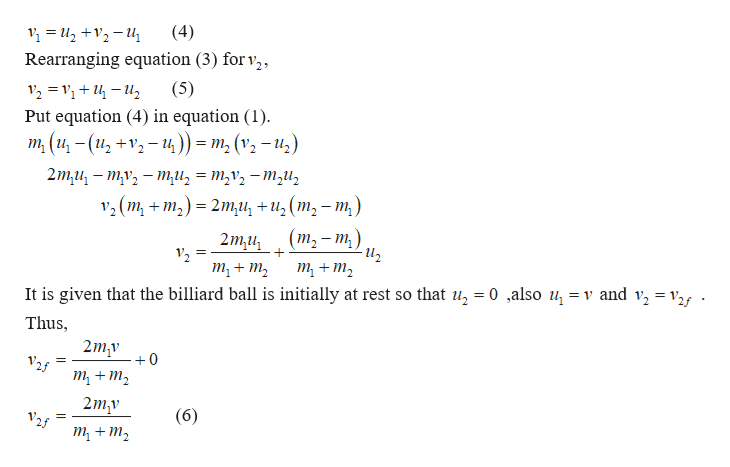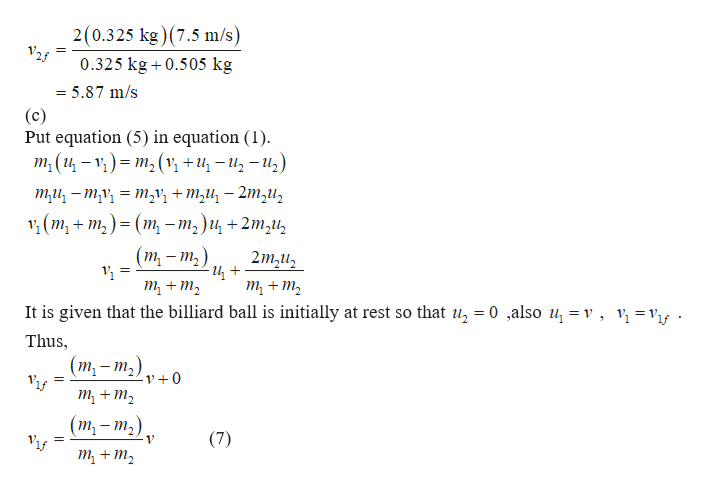# A cue ball of mass m1 = 0.325 kg is shot at another billiard ball, with mass m2 = 0.505 kg, which is at rest. The cue ball has an initial speed of v = 7.5 m/s in the positive direction. Assume that the collision is elastic and exactly head-on.(a)  Write an expression for the horizontal component of the billiard ball's velocity, v2f, after the collision, in terms of the other variables of the problem.(b)  What is this velocity, in meters per second?(c) Write an expression for the horizontal component of the cue ball's velocity, v1f, after the collision.(d)  What is the horizontal component of the cue ball's final velocity, in meters per second?

Question
114 views

A cue ball of mass m1 = 0.325 kg is shot at another billiard ball, with mass m2 = 0.505 kg, which is at rest. The cue ball has an initial speed of v = 7.5 m/s in the positive direction. Assume that the collision is elastic and exactly head-on.

(a)  Write an expression for the horizontal component of the billiard ball's velocity, v2f, after the collision, in terms of the other variables of the problem.

(b)  What is this velocity, in meters per second?

(c) Write an expression for the horizontal component of the cue ball's velocity, v1f, after the collision.

(d)  What is the horizontal component of the cue ball's final velocity, in meters per second?

check_circle

Step 1

(a)Since the collision is elastic, total linear momentum as well as the total kinetic energy of the system must be conserved.help_outlineImage TranscriptioncloseAssume the initial velocity of the cue ball to be u, , initial velocity of the billiard ball to be u, , final velocity of the cue ball to be v, and the final velocity of the billiard ball to be v, . The conservation of linear momentum gives, ти, + mи, — ту, + mу, m (и - ) — т, (у, - и,) (1) The conservation of kinetic energy gives 1 -тg 1 -тиз - 2 ()(-42) 1 1 vi) == m,(u?- -m2 (12 m (и, —)(и +r)- т, (и, —v,) (и, +,) (2) = Dividing equation (1) by (2), m (и, — v,) т, (и — ) (и, +т) т,(и,— т,) (и, +r,) т, (v, - и,) 1 1 (и,+v) (и, +7,) (3) fullscreen
Step 2

Rearranging equation (3)help_outlineImage Transcriptionclose(4) Rearranging equation (3) for v2 (5) Put equation (4) in equation (1) m (и, - (и, +r, — и,)) — т, (v, — и,) 2mи, — ту, — ти, — т,v, — т,и, 1 (m, + т,) - 2mи, +и,(т, - т,) (т, — т, 2mu т, + т, т + m, It is given that the billiard ball is initially at rest so that u, = 0 ,also u, = v and v, = v2f . Thus, 2mv +0 т + т, 2m,v т, + т, (6) fullscreen
Step 3

(b) Substitute the numerical v...help_outlineImage Transcriptionclose2(0.325 kg) (7.5 m/s) 0.325 kg 0.505 kg = = 5.87 m/s (c) Put equation (5) in equation (1) т, (и — ) - т,(v +u, - и, - и,) ти, — ту, — т,V, + m,u, — 2m,и, , (m, + т,) - (т, - т,)и, +2m,и, (т - т,) 2m2uz т + т, т + т, It is given that the billiard ball is initially at rest so that u, = 0 ,also u, = v , v\ =vif Thus, (т, - т,) -v +0 т, + т, (m, - т,). (7) т + т, fullscreen

### Want to see the full answer?

See Solution

#### Want to see this answer and more?

Solutions are written by subject experts who are available 24/7. Questions are typically answered within 1 hour.*

See Solution
*Response times may vary by subject and question.
Tagged in

### Physics